## 5.11 Final example

As a demonstration sampling schemes , consider taking a non-random sample of 10% of the pixels of an image (Fig. 5.3). What is the image? Seeing the big picture is hard using these non-random samples.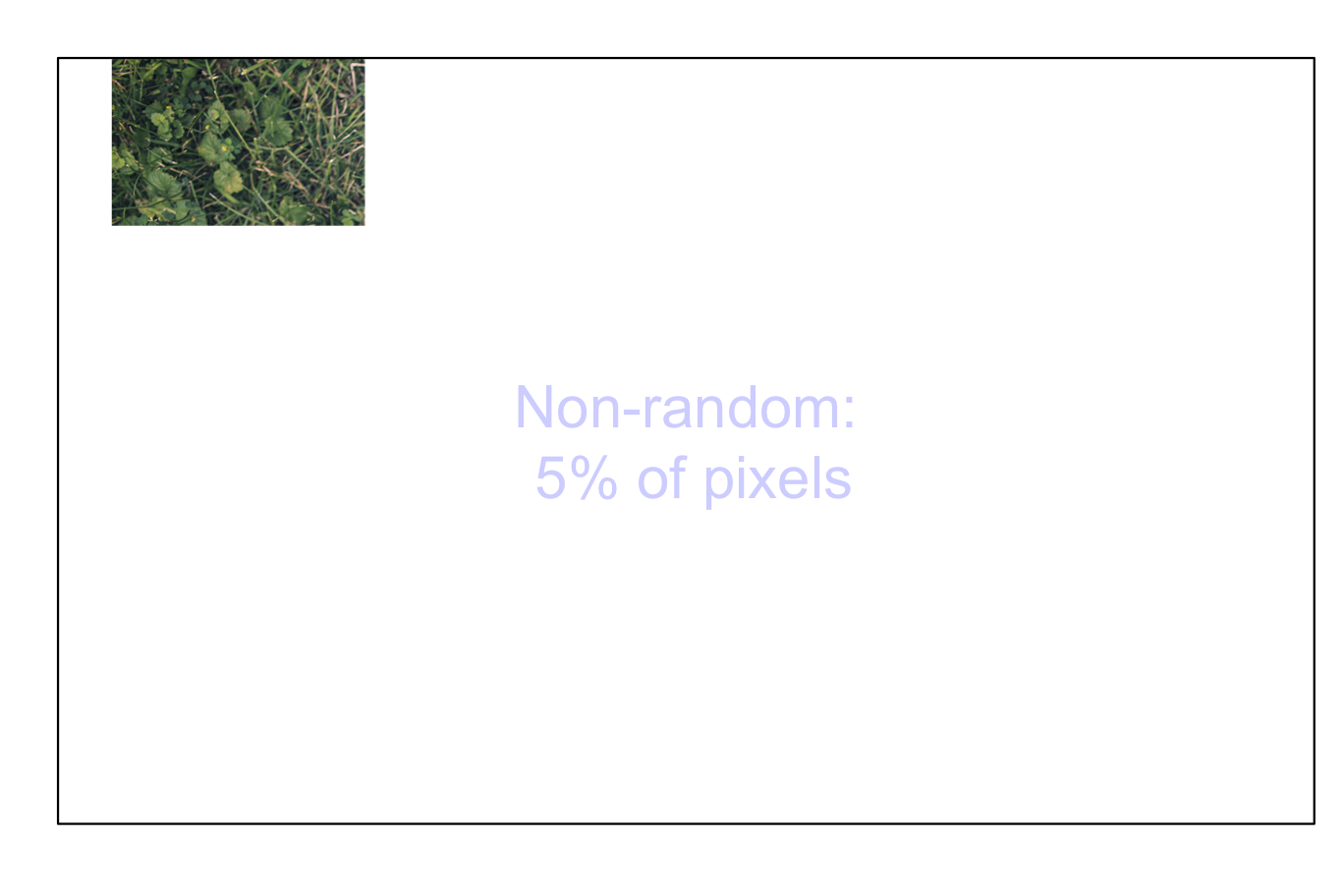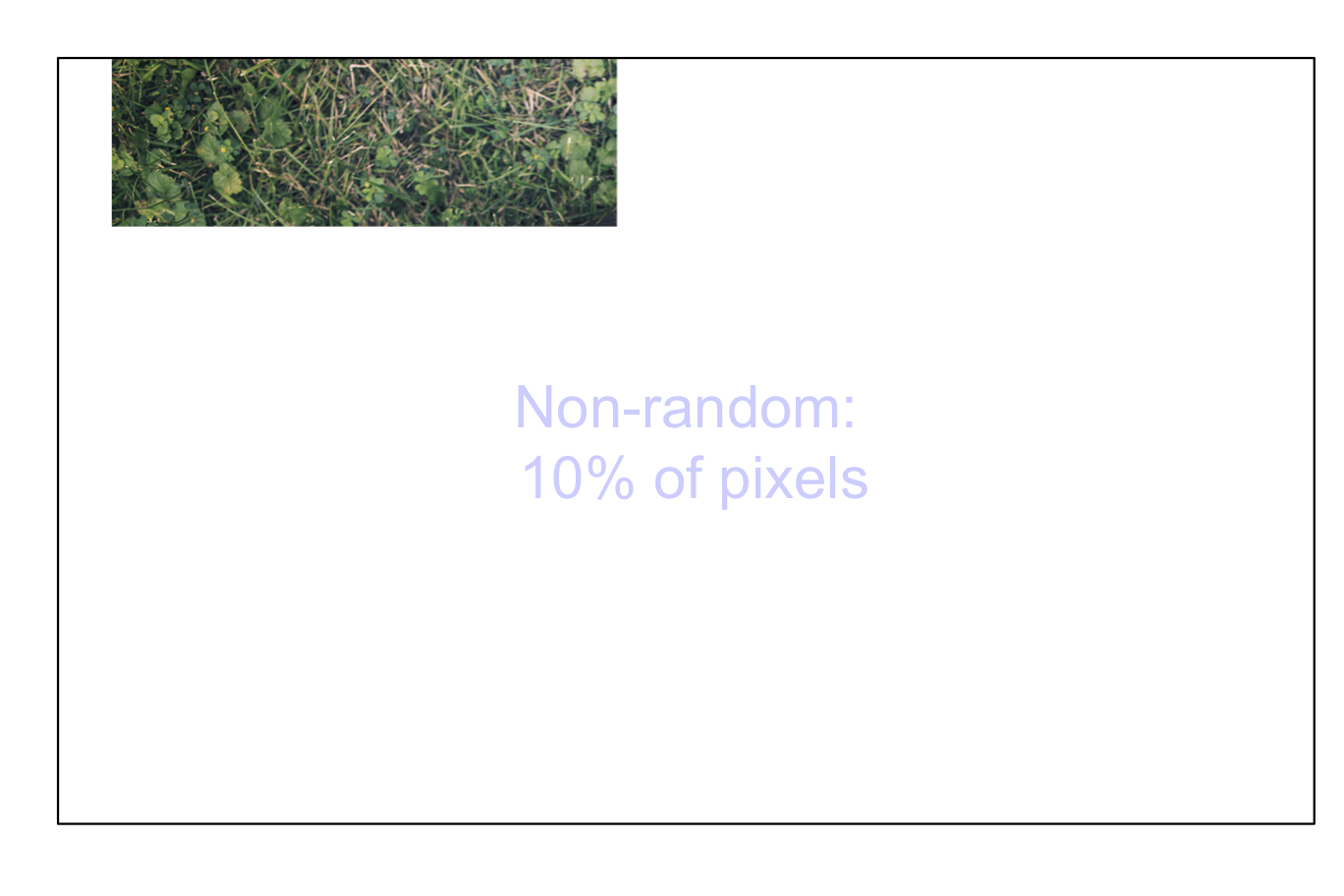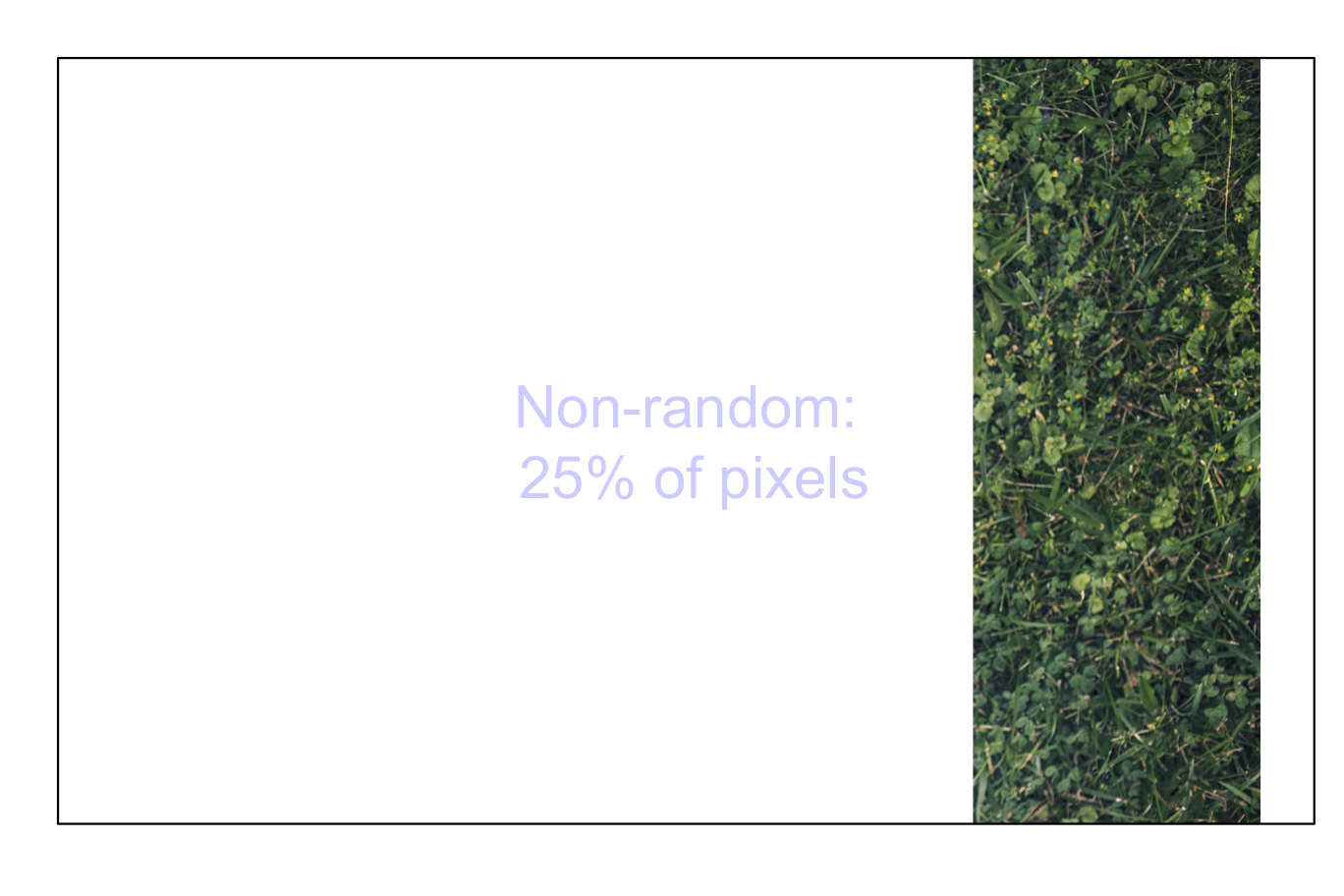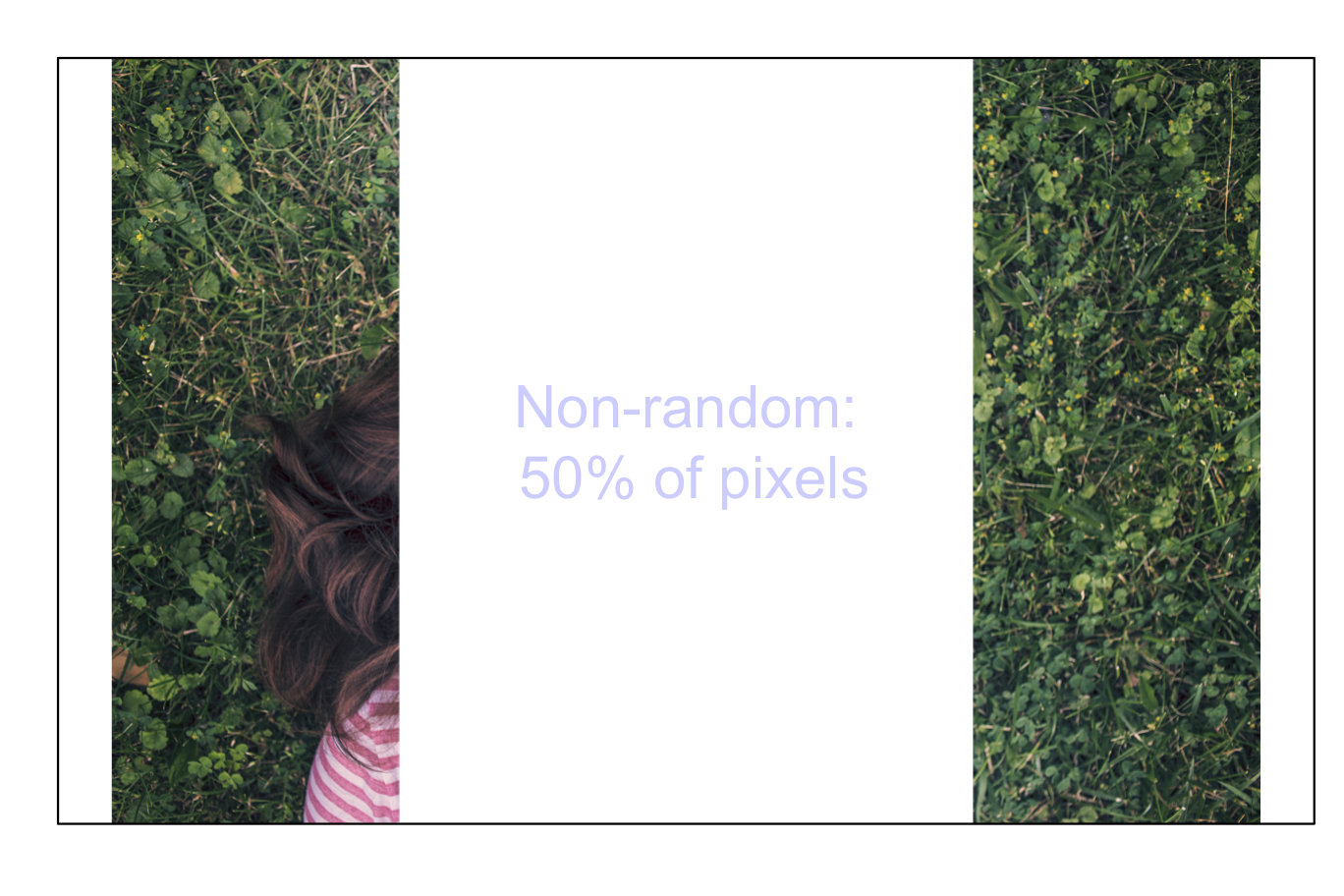FIGURE 5.3: Non-random samples from an image: 5 percent of pixels (top left); 10 percent of pixels (top right); 25 percent of pixels (bottom left); 50 percent of pixels (bottom right)

In contrast, taking simple random sample makes the big picture much clearer (Fig. 5.4).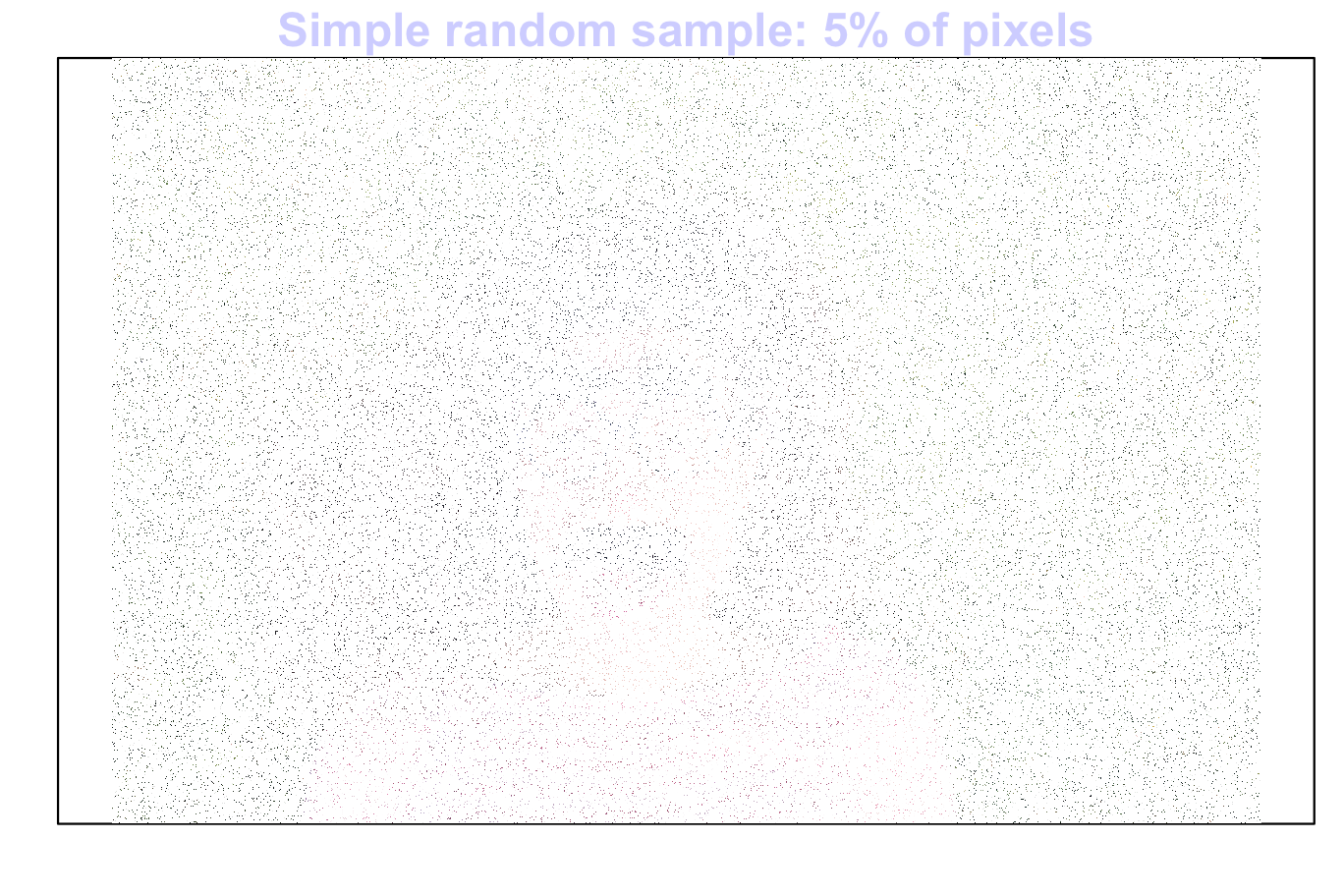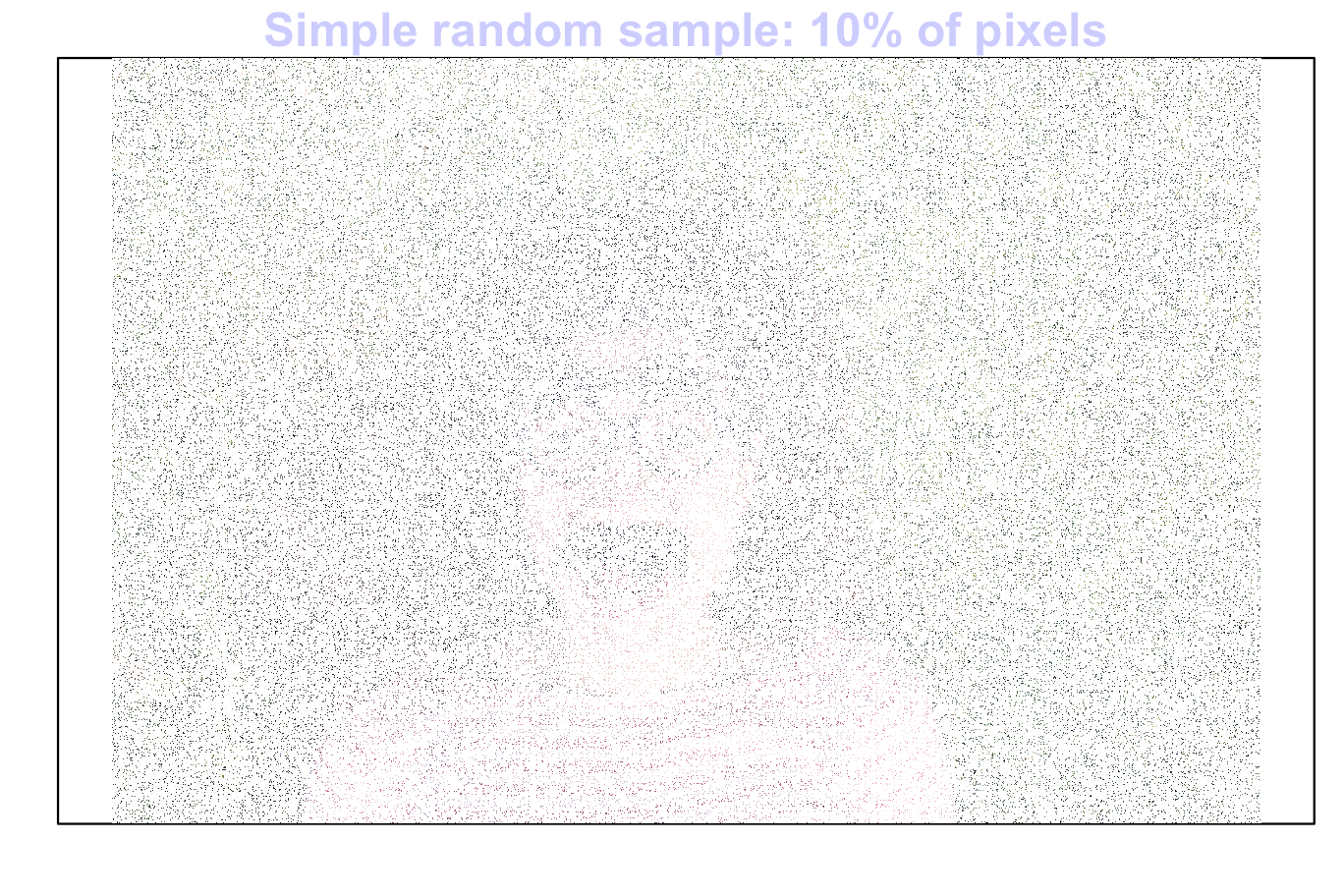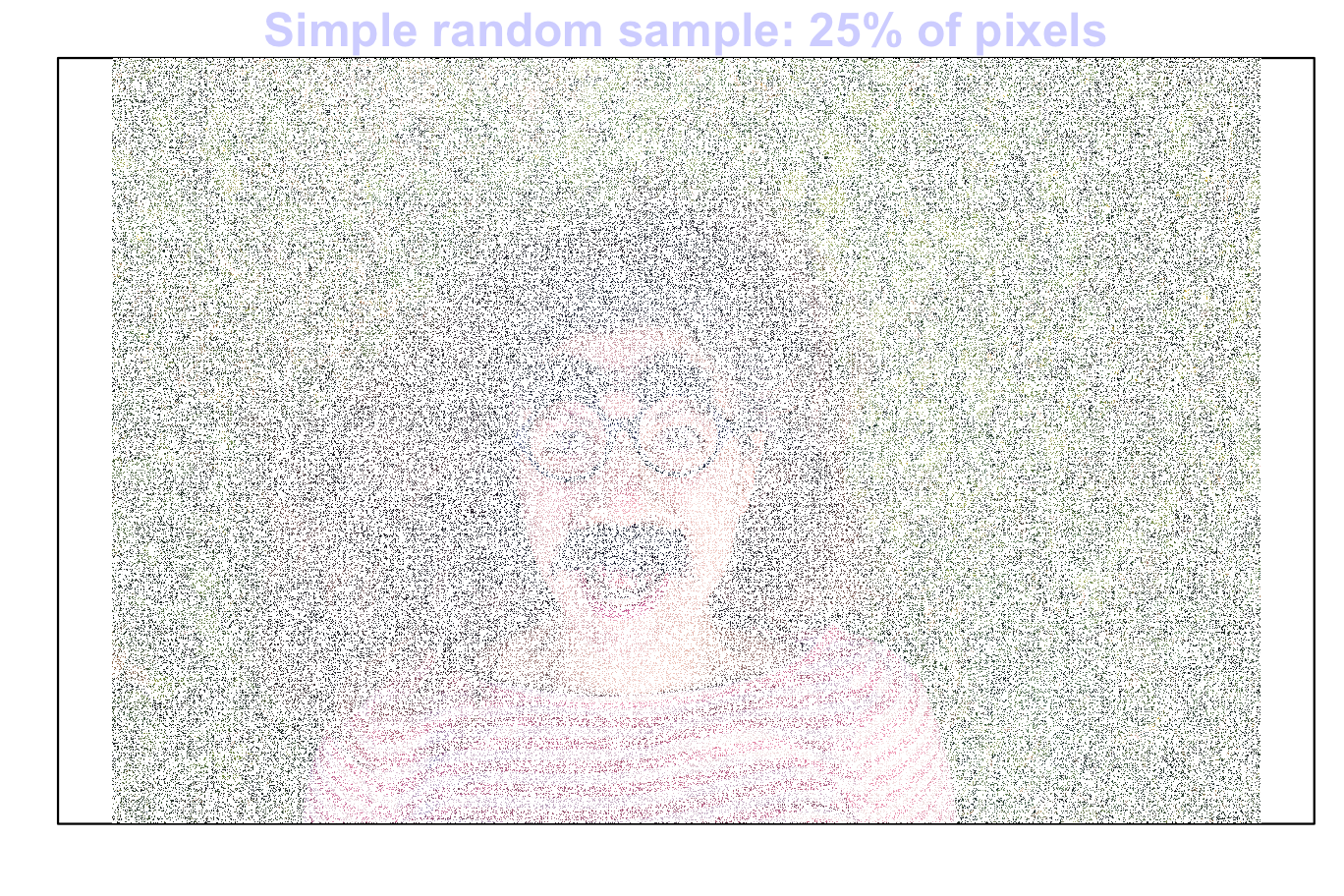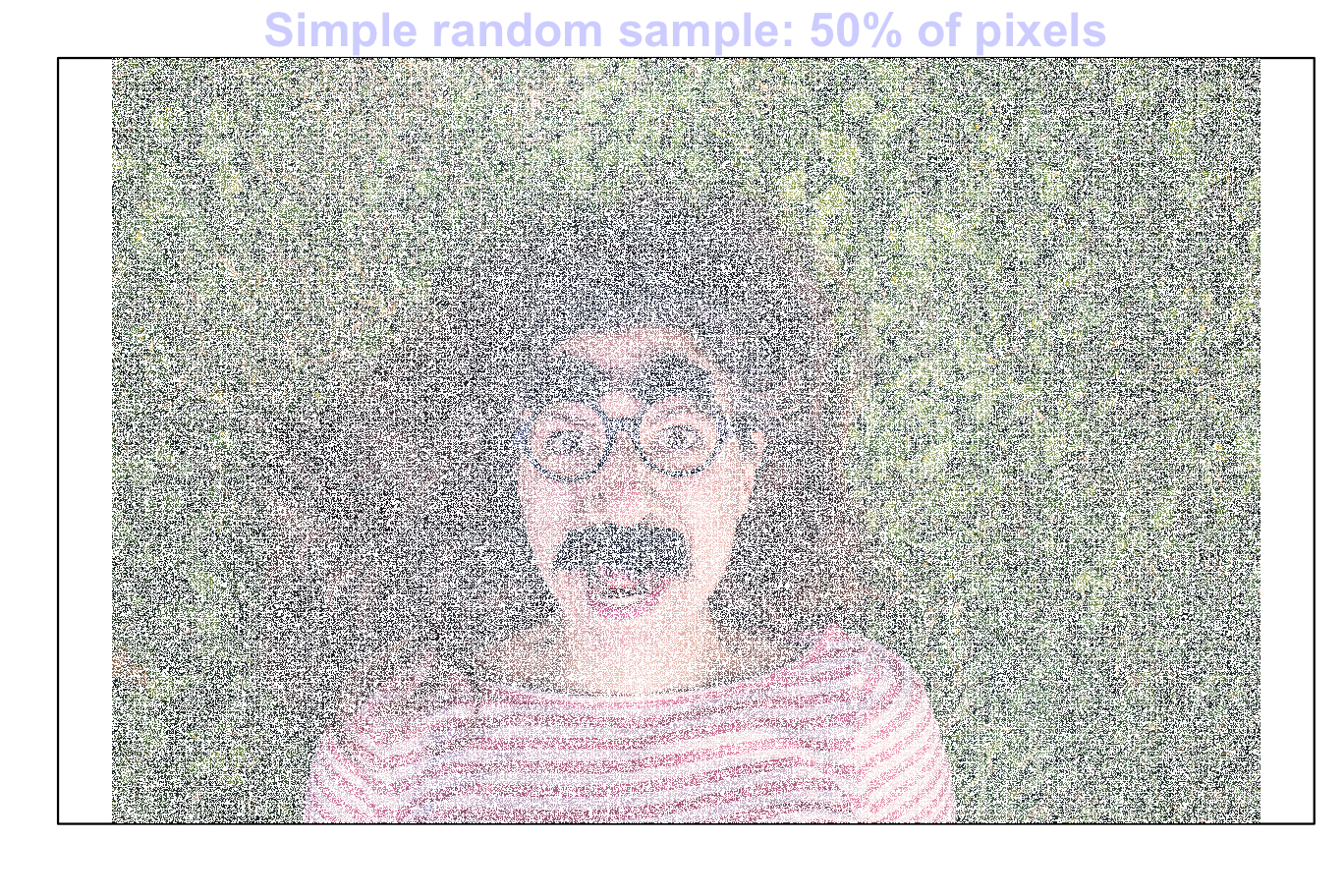FIGURE 5.4: Random samples from an image: 5 percent of pixels (top left); 10 percentof pixels (top right); 25 percent of pixels (bottom left); 50 percent of pixels (bottom right)

Indeed, any type of random sample makes seeing the big picture easier.

For example, for a cluster sample we treat each column as a cluster, and select some columns at random. Then, the entire chosen columns are selected.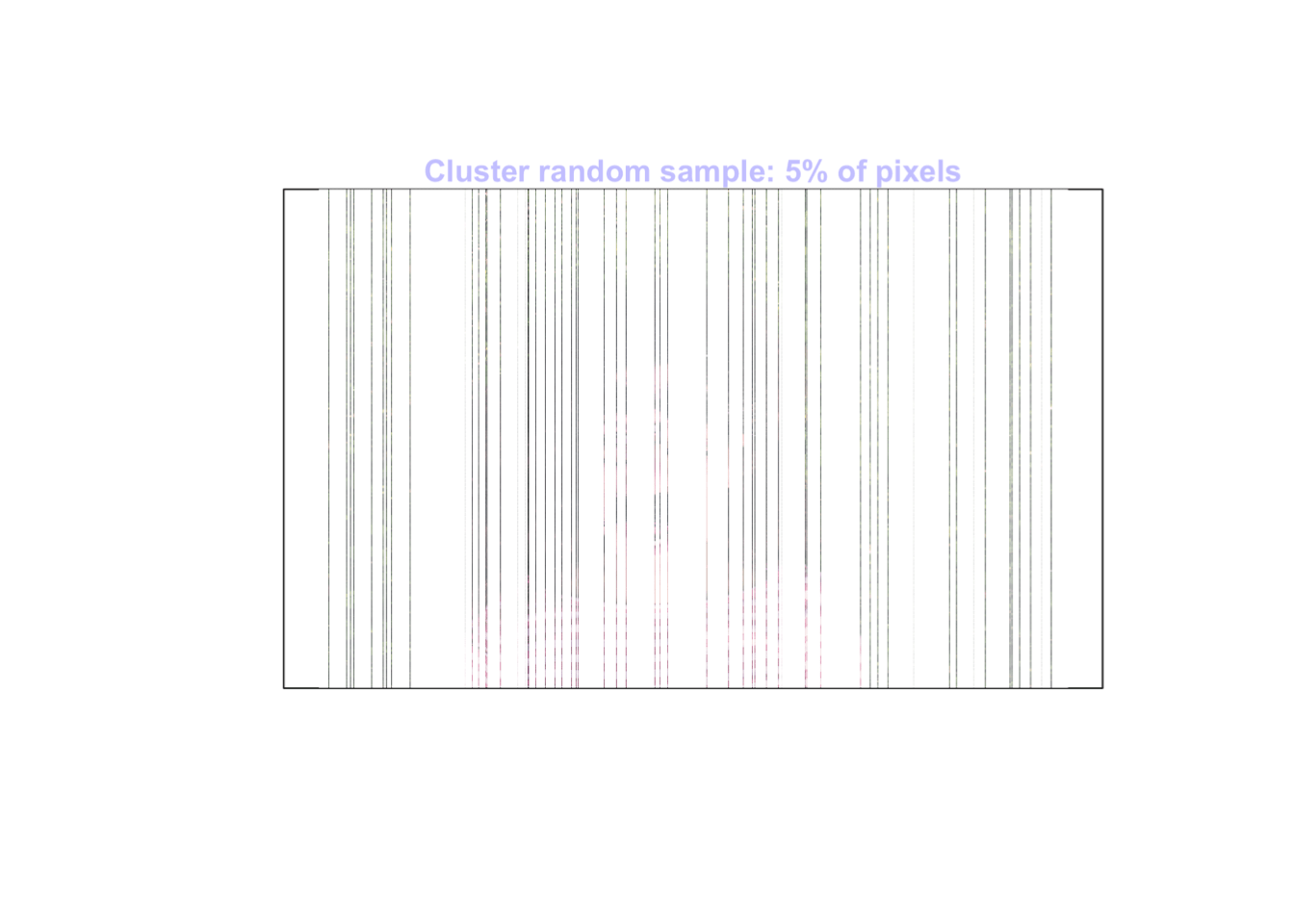For a systematic sample, we take:

• every 20th pixel for a 5% sample;
• every 10th pixel for a 10% sample;
• every 4th pixel for a 25% sample; and
• every second pixel for a 50% sample.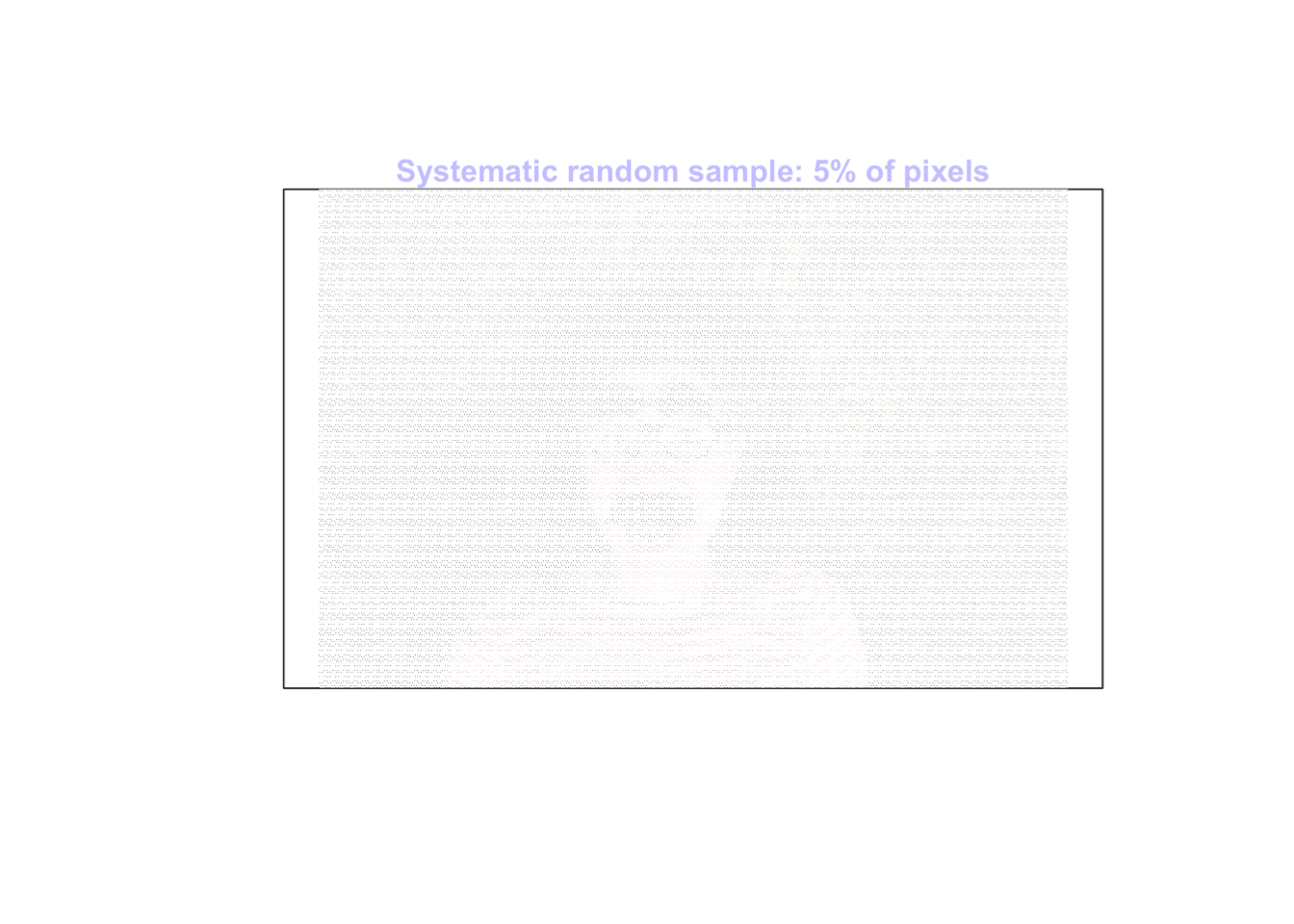For a multi-stage sample we select some columns at random, then select some pixels in those columns at random.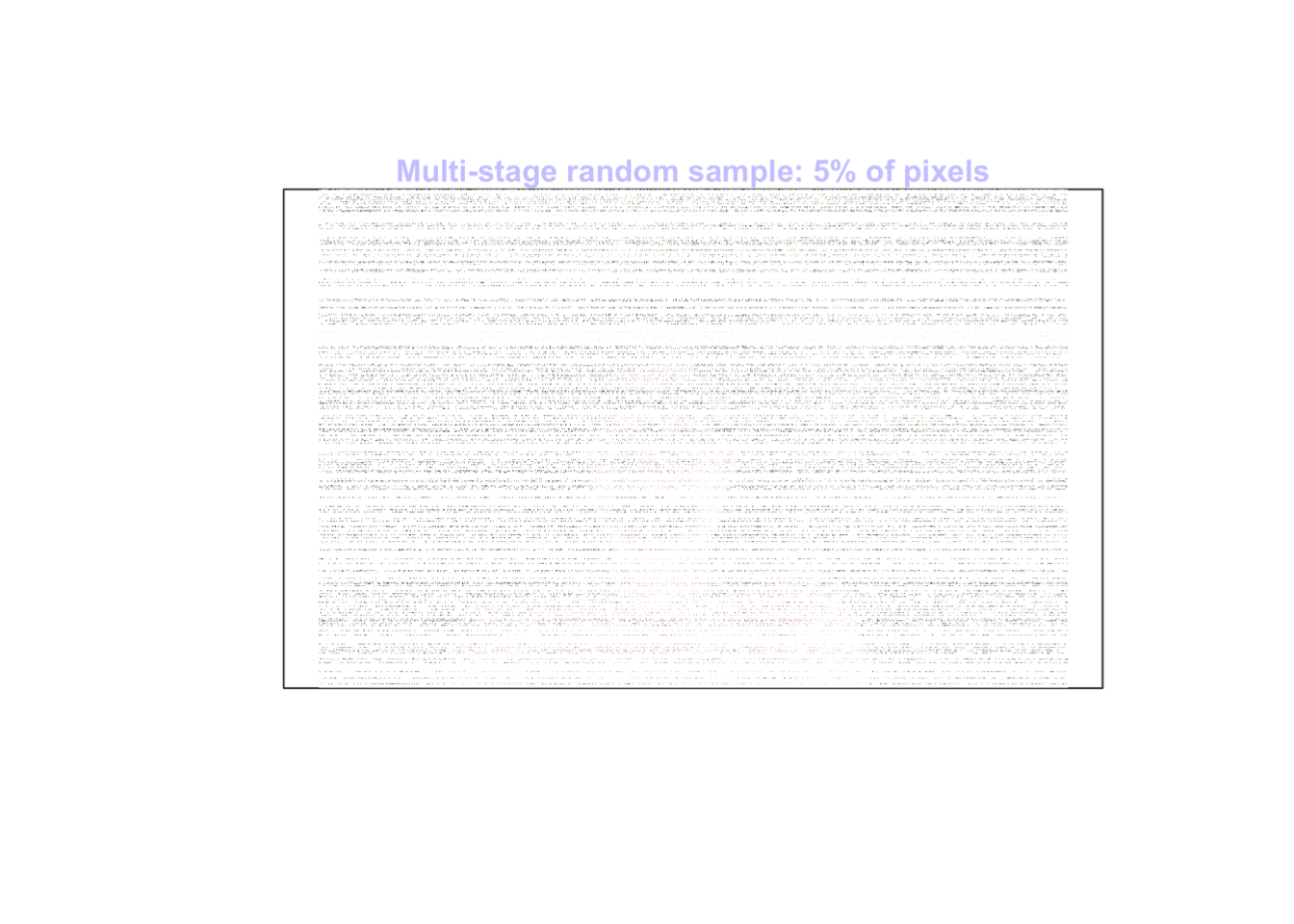For a stratified sample, we select :

• a simple random sample from the background greenery, and then
• a simple random sample from the person.

These two are then combined to get an overall random sample.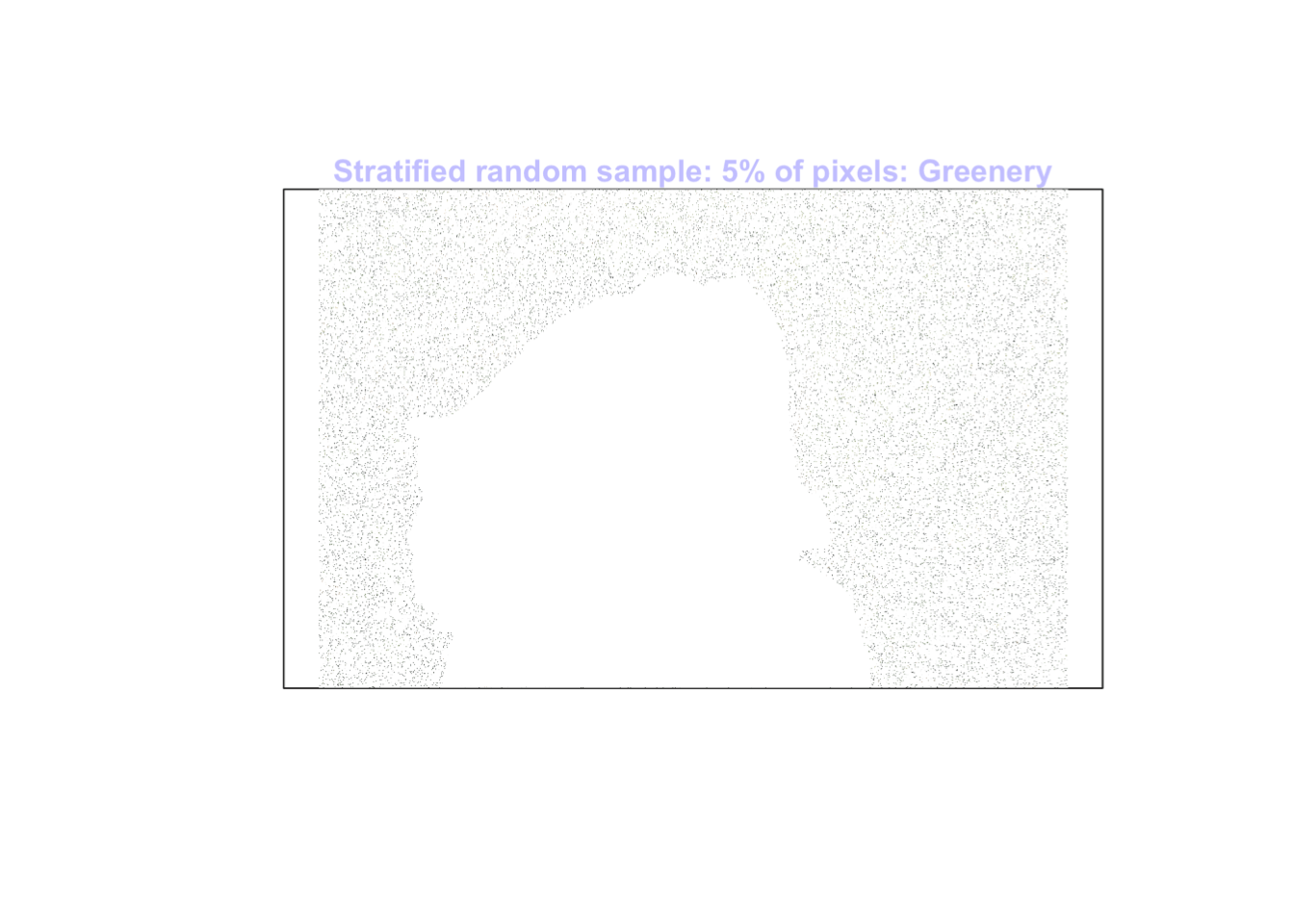### References

Marshman M, Dunn PK. Teaching statistics with experiential learning: A visual experience. Submitted;